# TypeError: 'numpy.ndarray' object is not callable in PythonLast updated: Jan 31, 2023
9 minIf you got the error "TypeError: 'Series' object is not callable", click on the second subheading.

## #TypeError: 'numpy.ndarray' object is not callable in Python

The Python "TypeError: 'numpy.ndarray' object is not callable" occurs when we try to call an array as if it were a function or use the same name for a variable and a function.

To solve the error, make sure to use square brackets when accessing an array element at a specific index, e.g. `my_array`.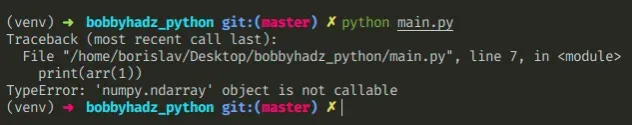Here is an example of how the error occurs.

main.py
```Copied!```import numpy as np

arr = np.array([1, 2, 3, 4])

# ⛔️ TypeError: 'numpy.ndarray' object is not callable
print(arr(1)) # 👈️ using parentheses
``````

The issue is that we used parentheses `()` instead of square brackets `[]` when accessing the array at a specific index.

To solve the error, use square brackets to access the array at an index.

main.py
```Copied!```import numpy as np

arr = np.array([1, 2, 3, 4])

# ✅ now using square brackets to access index
print(arr)  # 👉️ 1
print(arr)  # 👉️ 2
print(arr)  # 👉️ 3
print(arr)  # 👉️ 4
``````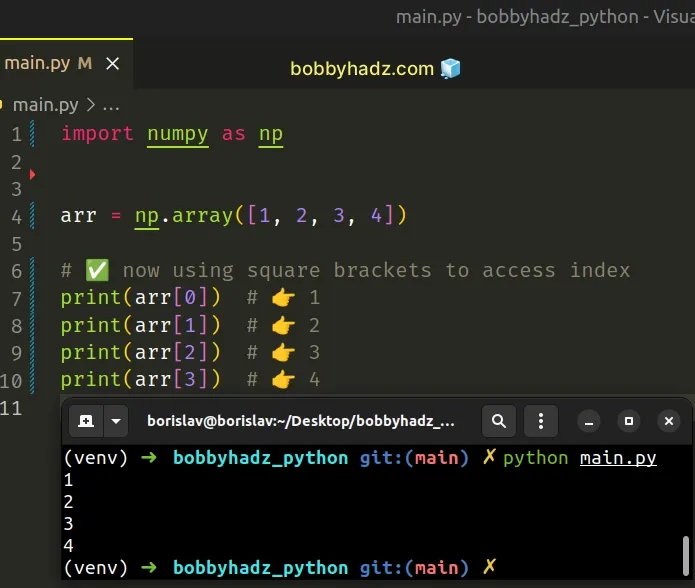Python indexes are zero-based, so the first item in an array has an index of `0`, and the last item has an index of `-1` or `len(arr) - 1`.

The syntax for array slicing is `arr[start:stop:step]`.

main.py
```Copied!```import numpy as np

arr = np.array([1, 2, 3, 4])

print(arr[0:2]) # 👉️ [1 2]
print(arr[1:3]) # 👉️ [2 3]
``````

The `start` index is inclusive and the `stop` index is exclusive (up to, but not including).

If the `start` index is omitted, it is considered to be `0`, if the `stop` index is omitted, the slice goes to the end of the list.

## #Common causes of the error

The error occurs for multiple reasons:

• Trying to access a numpy array with parentheses `()` instead of square brackets `[]`.
• Having a function and a variable with the same name.
• Overriding a built-in function by mistake and setting it to an array.
• Having a class method and a class property with the same name.
• Calling a function that returns a numpy array twice.

## #Having a function and a variable with the same name

Make sure you don't have a function and a variable with the same name.

main.py
```Copied!```import numpy as np

def example():

example = np.array([1, 2, 3, 4])

# ⛔️ TypeError: 'numpy.ndarray' object is not callable
example()
``````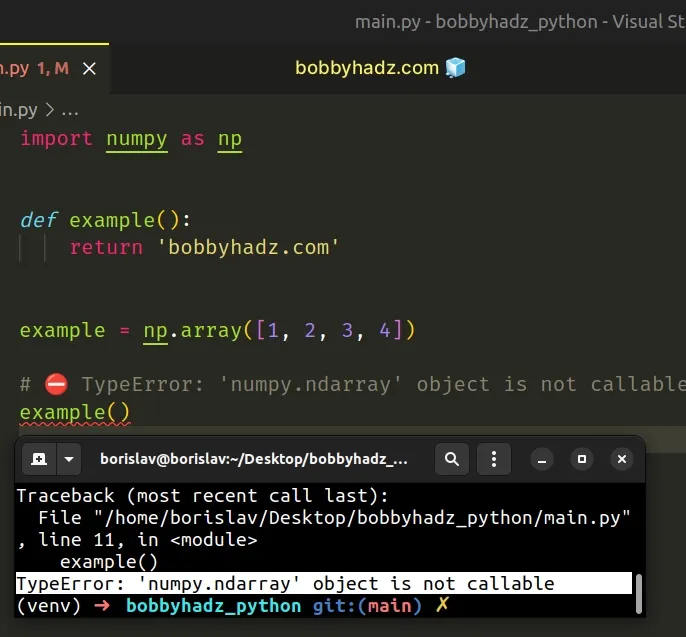The `example` variable shadows the function with the same name, so when we try to call the function, we actually end up calling the variable.

Renaming the variable or the function solves the error.

main.py
```Copied!```import numpy as np

def example():

arr = np.array([1, 2, 3, 4])

``````

We gave the variable a different name, so it no longer clashes with the function.

Now, we can call the function without any issues.

## #Calling an array as a function with parentheses

Make sure you aren't trying to call an array as a function in your code.

main.py
```Copied!```import numpy as np

arr = np.array([1, 2, 3, 4])

# ⛔️ TypeError: 'numpy.ndarray' object is not callable
arr() # 👈️ remove parentheses
``````

The code sample tries to call the numpy array as a function.

To resolve the issue, remove the parentheses or correct the assignment.

main.py
```Copied!```import numpy as np

arr = np.array([1, 2, 3, 4])

print(arr) # 👉️ [1 2 3 4]
``````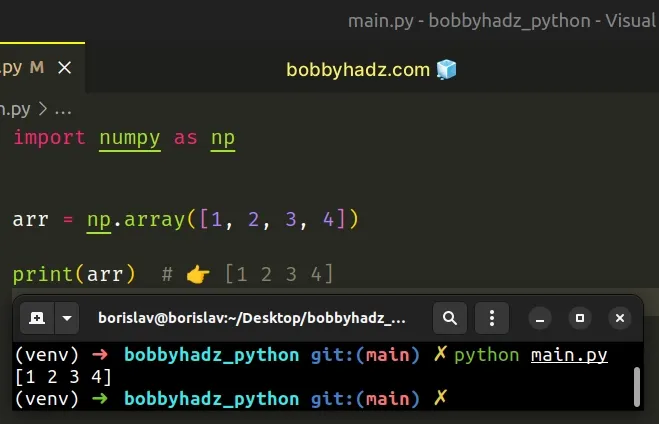The error also occurs if we override the classes and methods from the `numpy` module.

For example, don't import `array` from NumPy and declare a variable named `numpy` as it would shadow the official class.

## #Overriding a built-in function by mistake

Here is another example of how the error occurs.

main.py
```Copied!```import numpy as np

list = np.array([1, 2, 3, 4])

# ⛔️ TypeError: 'numpy.ndarray' object is not callable
list('abc')
``````

We declared a `list` variable and set it to a NumPy array.

The variable name clashes with the built-in `list` function, so when we try to call the function later on in our code, we actually call the NumPy array.

To solve the error, give the variable a different name that doesn't clash with built-in functions.

main.py
```Copied!```import numpy as np

a_list = np.array([1, 2, 3, 4])

print(list('abc')) # 👉️ ['a', 'b', 'c']
``````

The variable no longer shadows a built-in function, so the issue is resolved.

## #Calling a function that returns a NumPy array twice

Here is another example of how the error occurs.

main.py
```Copied!```import numpy as np

def get_array():
return np.array([1, 2, 3, 4])

# ⛔️ TypeError: 'numpy.ndarray' object is not callable
get_array()()
``````

Notice that we used two sets of parentheses when calling the `get_array` function.

The first set of parentheses calls the function and the function returns a NumPy array.

The second set of parentheses calls the NumPy array and causes the error.

To solve the error, remove the second set of parentheses.

main.py
```Copied!```import numpy as np

def get_array():
return np.array([1, 2, 3, 4])

print(get_array()) # 👉️ [1 2 3 4]
``````

Now we only call the function once and it returns a NumPy array.

## #Having a class method and a class property with the same name

If you are working with classes, make sure you don't have a method and a class property with the same name.

This causes the error in the same way as having a function and a variable with the same name does.

You have to rename the class method or the property, so they don't clash.

To solve the error, make sure:

• You aren't trying to access a NumPy array with parentheses `()` instead of square brackets `[]`.
• You don't have a function and a variable with the same name.
• You aren't overriding a built-in function and setting it to a NumPy array.
• You don't have a class method and a property with the same name.
• You aren't calling a function that returns a NumPy array twice.

## #TypeError: 'Series' object is not callable in Python

The Python "TypeError: 'Series' object is not callable" occurs when we try to call a `Series` object as if it were a function.

To solve the error, resolve any clashes between function and variable names and don't override built-in functions.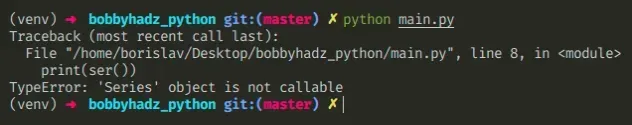Here is a very simple example of how the error occurs.

main.py
```Copied!```import pandas as pd

d = {'a': 1, 'b': 2, 'c': 3}

ser = pd.Series(data=d, index=['a', 'b', 'c'])

# ⛔️ TypeError: 'Series' object is not callable
print(ser())
``````

We are trying to call a pandas Series object as if it were a function.

## #Remove the parentheses to solve the error

If you added the parentheses by a mistake, simply remove them to solve the error.

main.py
```Copied!```import pandas as pd

d = {'a': 1, 'b': 2, 'c': 3}

ser = pd.Series(data=d, index=['a', 'b', 'c'])

# a    1
# b    2
# c    3
# dtype: int64
print(ser)
``````

We removed the parentheses and we are no longer trying to call the `Series` object as a function, so the issue is resolved.

## #Common causes of the error

The error occurs for multiple reasons:

• Trying to call a `Series` object with parentheses `()`.
• Having a function and a variable with the same name.
• Overriding a built-in function by mistake and setting it to a `Series` object.
• Having a class method and a class property with the same name.
• Calling a function that returns a `Series` object twice.

## #Having a function or variable that shadows a built-in function

Another common cause of the error is having a clash between function and variable names or overriding a built-in function.

main.py
```Copied!```import pandas as pd

d = {'a': 1, 'b': 2, 'c': 3}

ser = pd.Series(data=d, index=['a', 'b', 'c'])

# 👇️ this overrides the built-in list() function
list = ser

# ⛔️ TypeError: 'Series' object is not callable
print(list(['a', 'b', 'c']))
``````

We declared a variable named `list` and set it to a `Series` object.

This declaration overrides the built-in `list()` constructor, so when we try to call the `list()` constructor on the last line, we are actually calling the `Series` object.

To solve the error, rename the variable and not override any built-in methods.

main.py
```Copied!```import pandas as pd

d = {'a': 1, 'b': 2, 'c': 3}

ser = pd.Series(data=d, index=['a', 'b', 'c'])

# ✅ renamed variable
my_list = ser

print(list(['a', 'b', 'c']))
``````

The variable now has a different name and no longer shadows the built-in function.

## #Having a variable and a function with the same name

The error also occurs if you have a function and a variable that share the same name.

main.py
```Copied!```import pandas as pd

d = {'a': 1, 'b': 2, 'c': 3}

def series():

series = pd.Series(data=d, index=['a', 'b', 'c'])

# ⛔️ TypeError: 'Series' object is not callable
series()
``````

We defined a `series` variable after defining a function with the same name.

When we try to call the `series` function with parentheses, we are actually calling the `Series` object because the variable comes after the function.

To solve the error, rename your function or variable.

main.py
```Copied!```import pandas as pd

d = {'a': 1, 'b': 2, 'c': 3}

def my_func():

series = pd.Series(data=d, index=['a', 'b', 'c'])

``````

Now the function and variable no longer share the same name, so the issue is resolved.

## #When working with classes

If you are working with classes, make sure you don't have a method and a class property with the same name.

This causes the error in the same way as having a function and a variable with the same name does.

You have to rename the class method or the property, so they don't clash.

## #Calling a function that returns a Series object twice

Here is another example of how the error occurs.

main.py
```Copied!```import pandas as pd

def get_series():
d = {'a': 1, 'b': 2, 'c': 3}

ser = pd.Series(data=d, index=['a', 'b', 'c'])

return ser

# ⛔️ TypeError: 'Series' object is not callable
get_series()()
``````

Notice that we used two sets of parentheses when calling the function.

The first set calls the function and the function returns a `Series` object.

The second set of parentheses calls the `Series` object and causes the error.

To resolve the error, remove the second set of parentheses.

main.py
```Copied!```import pandas as pd

def get_series():
d = {'a': 1, 'b': 2, 'c': 3}

ser = pd.Series(data=d, index=['a', 'b', 'c'])

return ser

# a    1
# b    2
# c    3
# dtype: int64
print(get_series())
``````

A good way to start debugging is to `print(dir(your_object))` and see what attributes a `Series` object has.

main.py
```Copied!```import pandas as pd

d = {'a': 1, 'b': 2, 'c': 3}

ser = pd.Series(data=d, index=['a', 'b', 'c'])

print(dir(ser))
``````

If you pass a class to the dir() function, it returns a list of names of the class's attributes, and recursively of the attributes of its bases.

You probably meant to access an attribute using dot notation instead of call the `Series` as a function.

You can view the properties and methods a `Series` object has in the official pandas docs.

## #Conclusion

To solve the error, make sure:

• You aren't trying to call a `Series` object with parentheses.
• You don't have a function and a variable with the same name.
• You aren't overriding a built-in function and setting it to a `Series` object.
• You don't have a class method and a property with the same name.
• You aren't calling a function that returns a `Series` object twice.